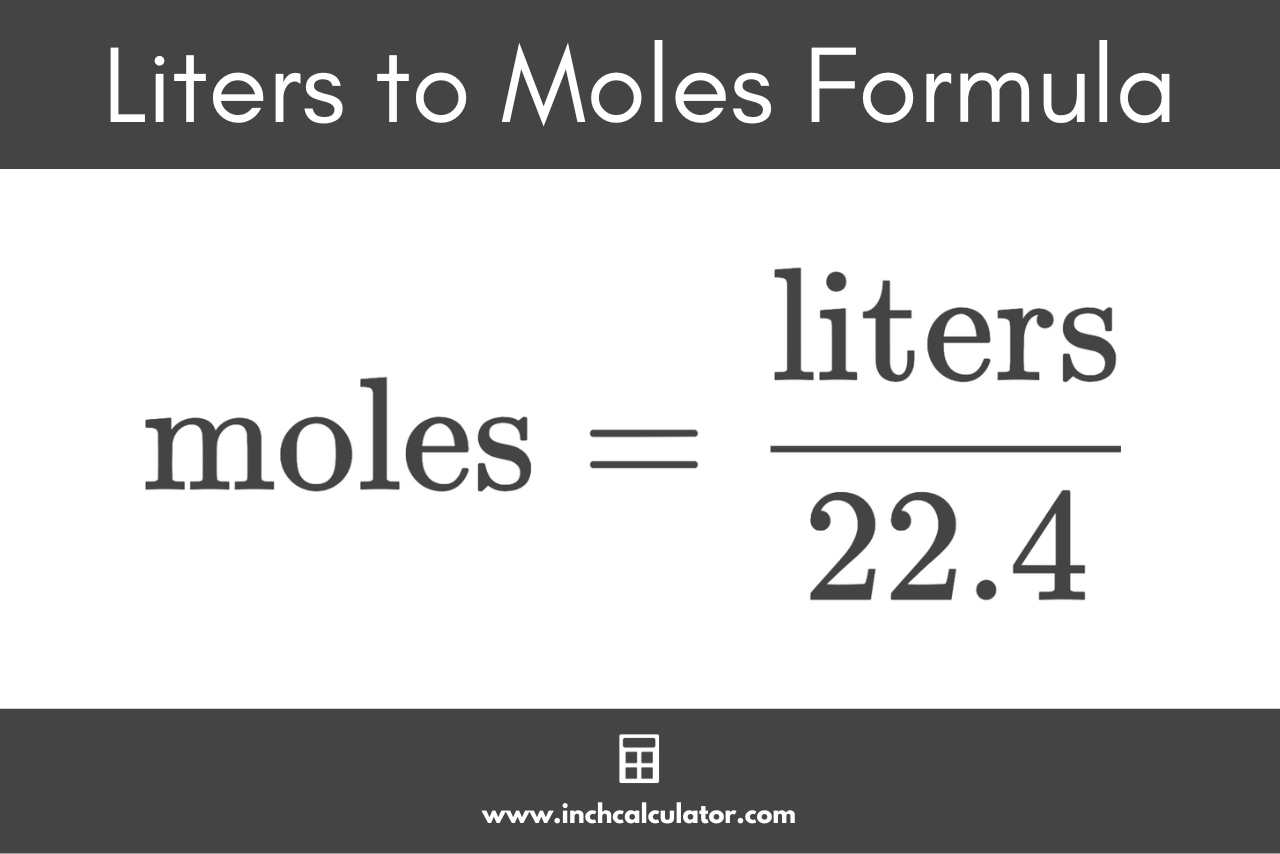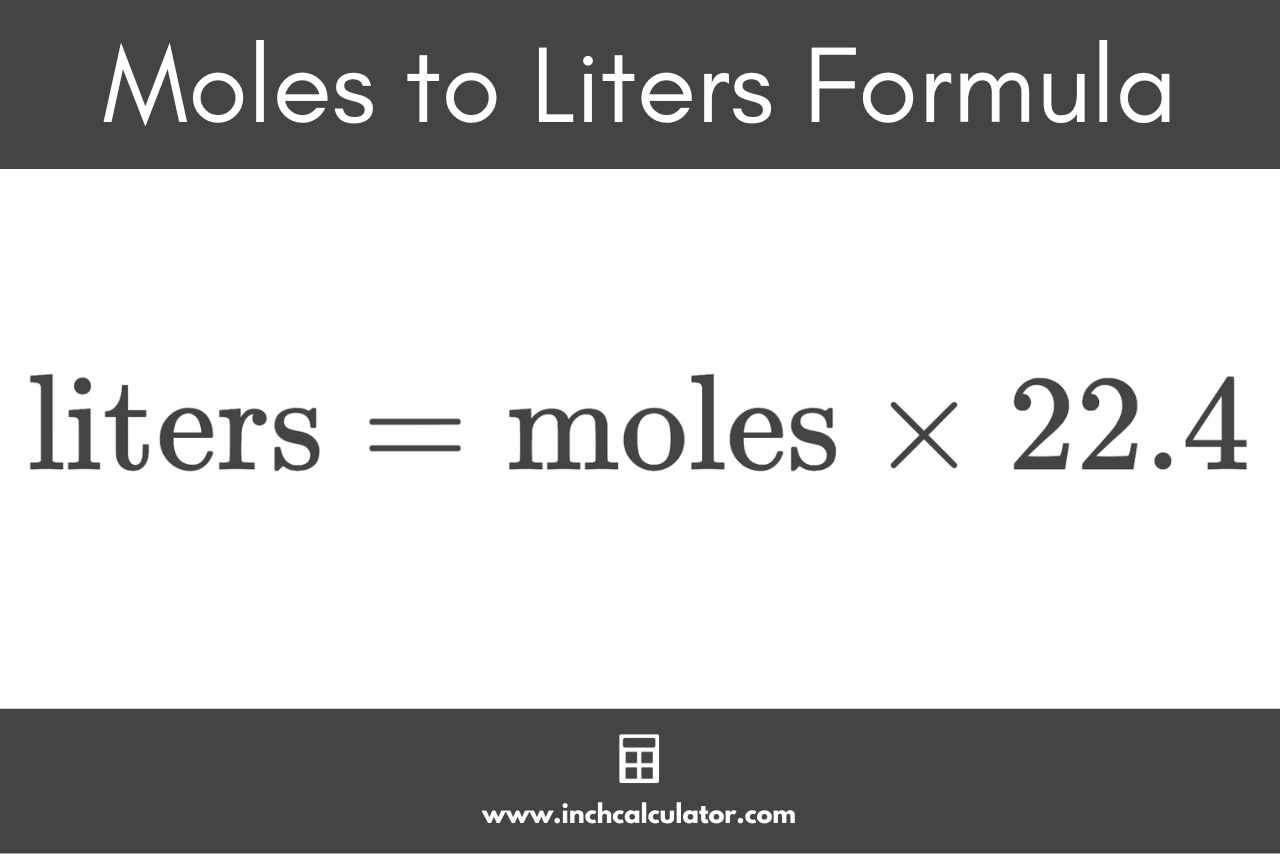# Liters to Moles Calculator

Convert liters to moles and vice-versa using the conversion calculator below.

moles

## Quantity:

moles
Learn how we calculated this below

## How to Convert Liters to Moles for Gasses

The SI base unit for the quantity of a substance is the mole, which is abbreviated mol, and a liter is an SI unit of volume equal to one cubic decimeter.

Whether you’re storing gasses in small tanks for chemistry experiments or large volumes in massive storage tanks for an industrial application, at some point, you may need to determine whether you have enough of a gas to carry out a chemical reaction.

To convert the volume of a gas in liters to the quantity of the gas in moles, you need to use the L to mol conversion formula for gasses.

### Liters to Moles Formula for Gasses

Unlike converting the mass in grams to moles, converting the volume of a gas in liters to moles uses a simple conversion formula that does not depend on the nature of the gas.

At standard temperature and pressure (STP), 1 mole of any ideal gas takes up 22.4 liters. Thus, the conversion factor used in the formula below is 22.4:

moles (mol) = liters (L) / 22.4

Thus, the amount of substance in moles is equal to the volume of ideal gas in liters divided by the conversion ratio of 22.4 L/mol.Did you know that you can convert atoms to moles using a similar formula?

## How to Convert Moles to Liters for Gasses

To convert moles to liters, you can reverse the formula and use the same conversion ratio.

liters (L) = moles (mol) × 22.4

Thus, the volume of a gas in liters at STP is equal to the amount in moles times the conversion ratio of 22.4 L/mol.## How Temperature and Pressure Affect the Volume of a Gas

Standard Temperature and Pressure (STP) are assumed in the conversions above using 22.4 L/mol. Standard temperature is assumed to be 0 degrees C, or 32 degrees F.

The pressure is assumed to be 1 atmosphere (abbreviated as atm), which is similar to air pressure at sea level.

However, you may be storing gasses in pressure tanks or cylinders, in which case the gas is compressed to conveniently store more of the gas in a smaller volume.

The temperature at which the gas is stored or the reaction is carried out also affects the volume of a gas in liters – at higher temperatures, a gas takes up more space. So higher pressure reduces the volume, while a higher temperature increases it.

## How to Convert Liters to Moles for Liquids

Many substances are dissolved in water or other solvents in a laboratory for later use in reactions. These solutions are typically described as “molar solutions.” For example, if you dissolve 1 mol of salt (NaCl) in 1 L of water (H2O), you have a 1M solution based on the number of moles per liter (mol/L).

If you dissolve 2 mol of salt in 1 L, then you have a 2M solution of salt in water.

### Liters to Moles Formula for Liquids

To convert liters to moles or moles to liters, you need to know the molarity (mol/L or M) of the solution, as described above. The conversion formula is:

moles (mol) = molarity (M) × liters (L)

So, if you have 4 L of a 2M solution, then you will have 8 mol of the substance.

## How to Convert Moles to Liters for Liquids

To convert moles to liters, you can reverse the formula:

liters (L) = moles (mol) / molarity (M)

For example, if you need 8 moles of a substance to carry out a reaction and in your laboratory, you have a 2M solution of that substance, you will need 4 L of the 2M solution to carry out the reaction.

## References

1. International Bureau of Weights and Measures, SI base unit: mole (mol), https://www.bipm.org/en/si-base-units/mole
2. National Institute of Standards and Technology, SI Units - Volume, https://www.nist.gov/pml/owm/si-units-volume